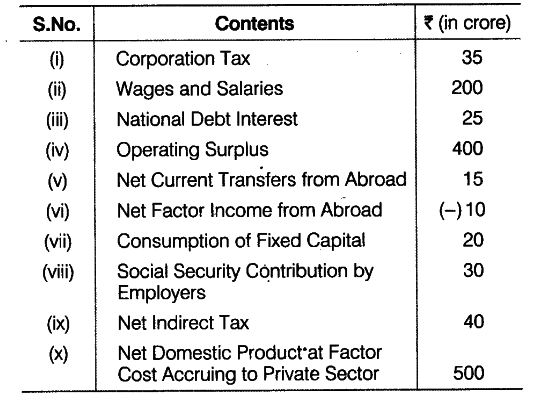# Calculate Personal income from the following data

Calculate (a) Gross National Product at Market Price and (b) Personal income from the following data:Net Domestic Product at Factor Cost (\$ND{{P}_{PC}}\$)
= Wages and Salaries + Social Security Contribution by Employers

• Operating Surplus = 200 + 30 + 400= Rs 630 crore
(a) Gross National Product at Market Price (GNPmp) = \$ND{{P}_{FC}}\$ + Consumption of Fixed Capital
• Net Indirect Tax + Net Factor Income from Abroad
= 630 + 20 + 40 + (-10) = Rs 680 crore
(b) Personal Income = Net Domestic Product at Factor Cost Accruing to Private Sector
• Net Factor Income from Abroad + National Debt Interest - Corporation Tax
= 500 + (-10) + (5 +15) + 25 - 35 = Rs 480 crore